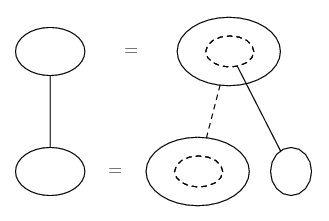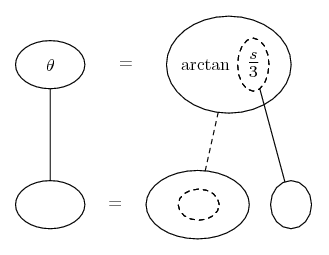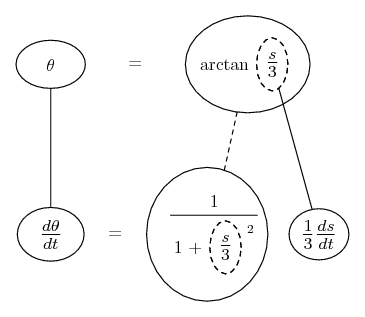# Thread: Related Rates Question

1. ## Related Rates Question

I have no idea how to start with this problem. I have the general outline but I do not know how to solve it. By general outline I mean the sketch of the problem.

A lighthouse is located on a small island 3 km away from the nearest point P on a straight shoreline and its light makes four revolutions per minute. How fast is the beam of light moving along the shoreline when its 1km from P?

If anyone can help on how to go about this problem it would be much appreciated. Thanks in advance.

2. Related rates nearly always depend on the chain rule, so you might want to try filling out this pattern...... where straight continuous lines differentiate downwards (integrate up) with respect to the main variable (in this case time), and the straight dashed line similarly but with respect to the dashed balloon expression (the inner function of the composite which is subject to the chain rule).

So what have we got here? theta the angle, d/dt of theta = 8 pi = rate of increase in theta, s = 1 = the opposite side of the right triangle i.e. the distance of the beam from P, 3 the length of the adjacent = distance of the lighthouse from P, ds/dt the rate of increase of s, and arctan getting theta from s and 3, as here...So differentiate with respect to the inner function, and the inner function with respect to t ...

Spoiler:... and sub in the given values of s and d/dt of theta

__________________________________________
Don't integrate - balloontegrate!
Balloon Calculus: Gallery
Balloon Calculus Drawing with LaTeX and Asymptote!

3.Originally Posted by kevinmI have no idea how to start with this problem. I have the general outline but I do not know how to solve it. By general outline I mean the sketch of the problem.

A lighthouse is located on a small island 3 km away from the nearest point P on a straight shoreline and its light makes four revolutions per minute. How fast is the beam of light moving along the shoreline when its 1km from P?

If anyone can help on how to go about this problem it would be much appreciated. Thanks in advance.
Draw a picture. In particular, draw a line from the light house to P- that line will be perpendicular to the shore. Drawing a line from the light house to the point x km from P, along with the shore, gives a right triangle with "opposite side" of length x and "near side" of length 3. $\displaystyle tan(\theta)= x/3$.

Differentiate both sides of that with respect to t (use the chain rule: $\displaystyle \frac{df(\theta)}{dt}= \frac{df(\theta)}{d\theta}\frac{d\theta}{dt}$) to get an equation relating $\displaystyle \frac{dx}{dt}$ and $\displaystyle \frac{d\theta}{dt}$.

4. When I differentiated both sides I got 1/cos^2(theta) x d(theta)/dt = 1/3

is that correct?

The next step in this problem would to be substitute in the variables such as the d(theta)/dt = 8pie radians/sec and isolate theta by using inverse cosine?

5. the anwser i got was 83.78 km/min

6. correct

### relative rates rotating lighthou

Click on a term to search for related topics.

#### Search Tags

question, rates, related